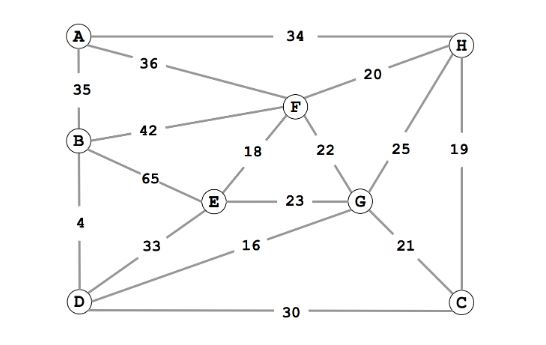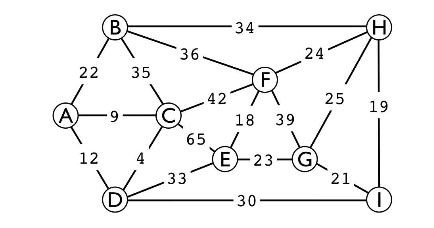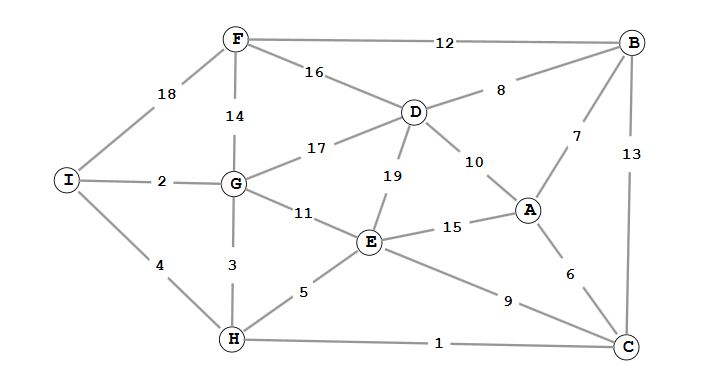### MINIMUM SPANNING TREES STUDY GUIDE

Definition of edge-weighted graph and MST.

• What is a spanning tree?
• What is a minimum spanning tree?
• How many edges are in a (minimum) spanning tree of a graph with V vertices?
• Why do we make the simplifying assumptions that the graph is connected and the edge weights are distinct?

Cuts.

• What is a cut?
• How many cuts are there for a graph with V vertices?
• Cut property: given a cut, the crossing edge of minimum weight belongs to the MST. This is the most important point of this lecture, and you should be very familiar with its proof.

Manually performing Kruskal's and Prim's algorithms. These are easy and you should be able to do them even under the influence of powerful sedatives.

Why Kruskal's and Prim's algorithms work.

• Kruskal's algorithm is a special case of the generic greedy algorithm.
• Prim's algorithm is a special case of the generic greedy algorithm.

Implementing Kruskal's and Prim's algorithms.

• What sort of data do we track in Kruskal's algorithm, and which data structures do we use to track this data?
• What is the running time for typical implementations of these data structures?
• What sort of data do we track in Lazy Prim's algorithm, and what data structures do we use to track this data?
• What is the running time for typical implementations of these data structures?
• For Eager Prim's, why do we track vertices instead of edges? What does this do to our space requirements?
• What is the special data structure that we use to track vertices and their best known distance?
• How do we change the priority of a vertex using this special data structure (you should be able to give an example of a method call)?

### Recommended Problems

#### C level

1. Consider the following weighted graph.Give the list of edges in the MST in the order that Kruskal's algorithm includes them.
Give the list of edges in the MST in the order that Prim's algorithm includes them. Start Prim's algorithm from vertex A. Answers
2. Consider the following undirected weighted graph.Give the list of edges in the MST in the order that Kruskal's algorithm includes them.
Give the list of edges in the MST in the order that Prim's algorithm includes them. Start Prim's algorithm from vertex F. Answers
3. Consider the following undirected weighted graph.Give the list of edges in the MST in the order that Kruskal's algorithm includes them.
Give the list of edges in the MST in the order that Prim's algorithm includes them. Start Prim's algorithm from vertex A. Answers
4. Spring 12 Final, #4a, #4b
5. Would Kruskal's or Prim's algorithm work with edge-weighted digraphs?

#### B level

1. Using the weighted undirected graph from C level #3, suppose that the edge D - I of weight w is added to the graph. For which values of w is the edge D - I in a MST? Answers
2. Fall 12 Final, #4a, #4b, #4c, #4d
3. Textbook 4.3.8 - this is a particularly handy concept!
4. Textbook 4.3.12
5. Textbook 4.3.13
6. Textbook 4.3.20
7. True or False: Given any two components that are generated as Kruskal's algorithm is running (but before it has completed), the smallest edge connecting those two components is part of the MST.
8. Textbook 4.3.19 - to be clear, for each vertex v, we maintain edgeTo[v] and distTo[v] and to find the nontree vertex with the smallest distTo[], we scan through the entire distTo[] array.

#### A level

1. You are given an edge-weighted undirected graph, using the adjacency list representation, together with the list of edges in its minimum spanning tree (MST). Describe an efficient algorithm for updating the MST, when each of the following operations is performed on the graph. Assume that common graph operations (e.g., DFS, BFS, finding a cycle, etc.) are available to you, and don't describe how to re-implement them.
1. Update the MST when the weight of an edge that was not part of the MST is decreased. Give the order-of-growth running time of your algorithm as a function of V and/or E.
2. Update the MST when the weight of an edge that was part of the MST is decreased. Give the order-of-growth running time of your algorithm as a function of V and/or E.
3. Update the MST when the weight of an edge that was not part of the MST is increased. Give the order-of-growth running time of your algorithm as a function of V and/or E.
4. Update the MST when the weight of an edge that was part of the MST is increased. Give the order-of-growth running time of your algorithm as a function of V and/or E.